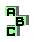EULER LINE    (1/8)

 r20 first Brocard triangle, centroid, Tarry point r28 Fermat points, centroid r35 isodynamic points, Fermat points, centroid r36 isodynamic points, Fermat points, Napoleon points, symmedian point, harmonic points r39 orthocentroidal circle, Fermat axis r52 Carnot triangle, Gossard triangle, medial triangle , antimedial triangle, homothetic triangles d Evans point d Gossard triangle d Kiepert parabola d Schiffler point d Parry reflection point d point X(110) d Tixier point d point X(74) d point X(647) d point X(376) d points X(1113)-X(1114) r81 Jerabek hyperbola, isogonal points r84 point X(125), orthopole r110 Stammler hyperbola r117 tripolar, inconic r122 point X(110), Simson line r124 point X(110), circumcenter, perspective, similar triangles r125 altitude feet, point X(125)1 2 3 4 5 6 7 8